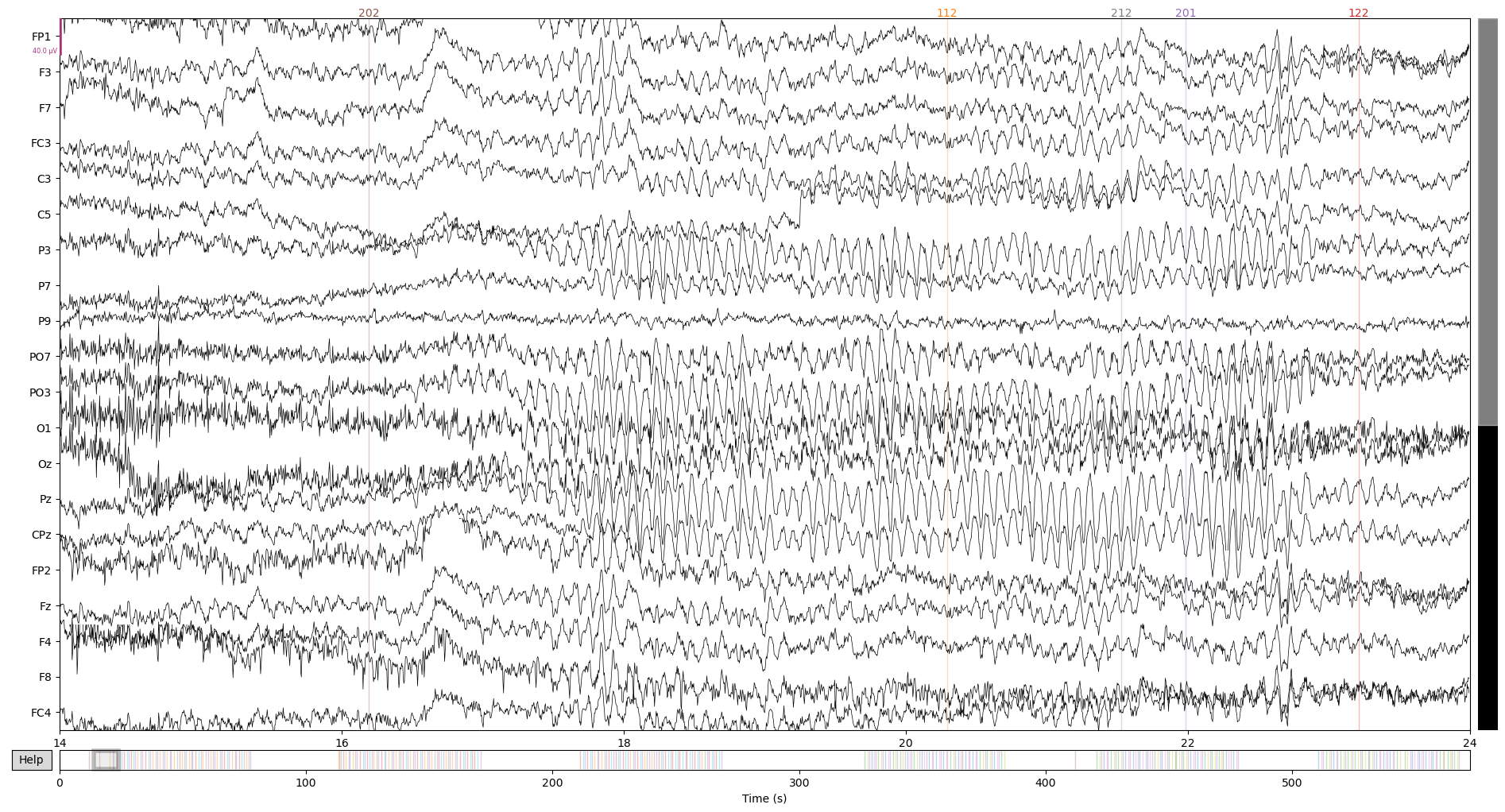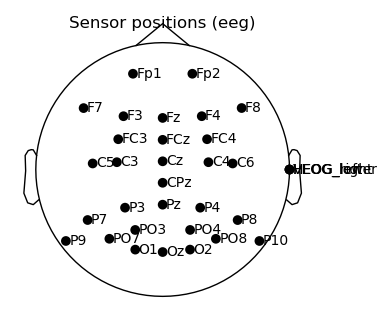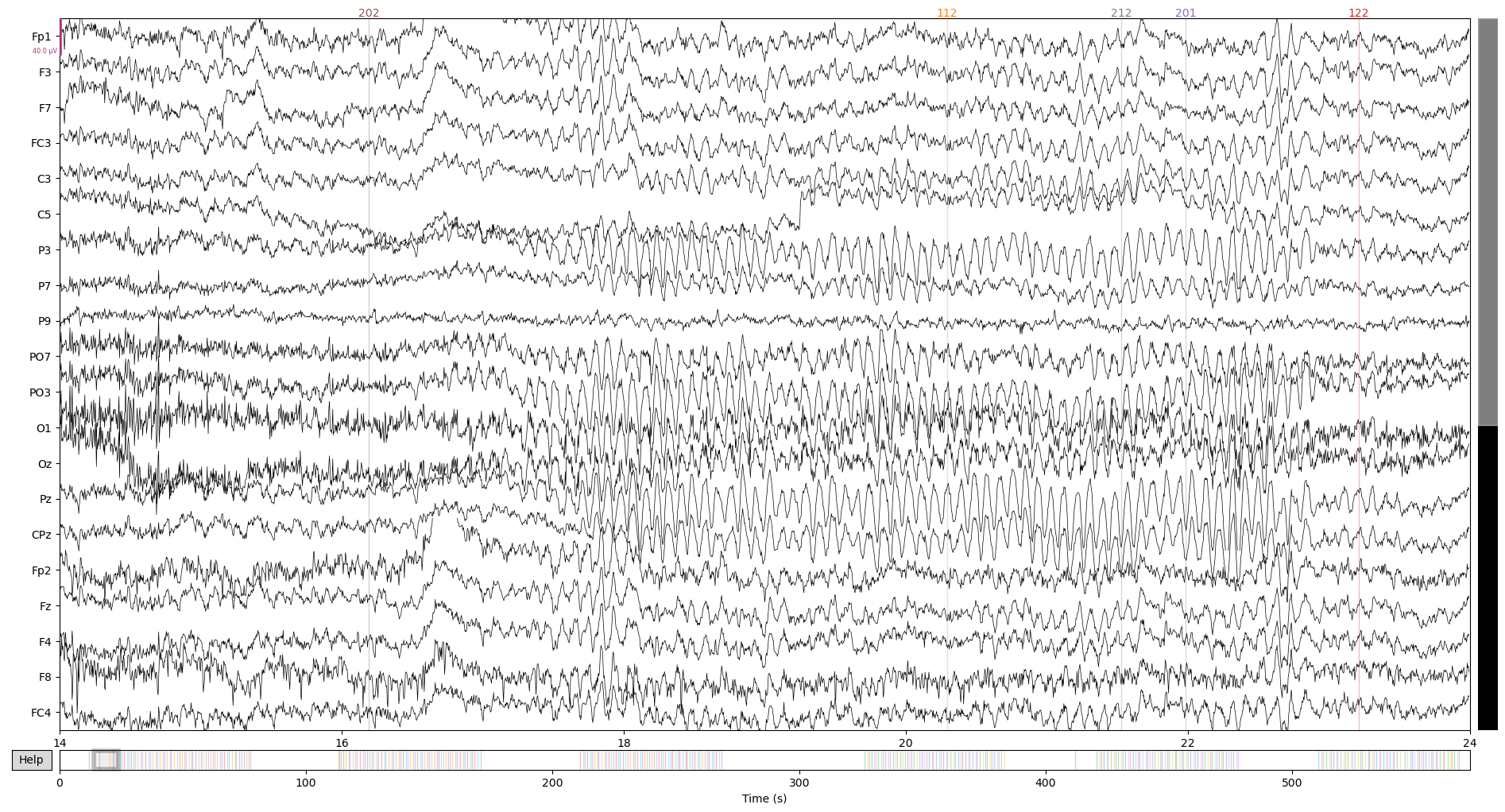# ERP Core N400 preprocessing in MNE-Python

Steven Luck is one of the greatest authorities in EEG data processing and analysis. His lab has lauched the ERP Core database with how-to preprocess and get some of the most studied ERP waveforms in cognitive neuroscience.

Luck’s pipelines are available as Matlab scripts to be run with EEGLAB and ERPLAB toolboxes. Here, I adapt the N400 ERP pipeline to MNE-Python, an open-source alternative to conduct EEG analyses. The idea is to show how MNE-Python works while replicating the pipeline proposed in ERP CORE. The idea is not to teach EEG preprocessing, but I hope this material can help people considering to switch their EEG analyses from MATLAB to Python and also newcomers.

I will walkthrough each of N400 ERP CORE’s scripts. In this first part, I will cover all the proprocessing steps. I’ll always show do the steps in the data of a single participant and finish with a loop to run all subjects. ERP CORE Data and scripts can be found here at OSF.

Before we start, we load the libraries we will use, libraries are similar to MATLAB’s toolboxes. The most important is MNE-Python, of course. But we also load useful libraries to work with data in general: NumPy and Pandas.

# set working directory
import os
os.chdir('D:\\EEGdata\\Erp CORE\\N400')

import mne
# import some methods directly so we can call them by name

import numpy as np
import pandas as pd

# graphics definitions for jupyter lab
import matplotlib.pyplot as plt
%matplotlib qt
%gui qt


## Individual-Subject EEG and ERP Processing Procedures

### Script 1: load, reference, downsample, montage and filter

These steps are in the Import_Raw_EEG_Shift_DS_Reref_Hpfilt.m script of ERP CORE.

To start: load data, identify events (or “triggers”), downsample data do 256Hz, change reference to mastoids and create H/VEOG channels.

In MNE, events are loaded as annotations. Annotations are identifiers associated to the data. They can be useful to show experiment sections in plots and to mark noisy segments in the data.

subj = 1
raw_name = '{0}\\{0}_N400.set'.format(subj)

#Annotations. Uncomment if you want to  see the structure
#set(raw.annotations.description)
#set(raw.annotations.duration)
#raw.annotations.onset

#Shift the stimulus event codes forward in time to account for the LCD monitor delay
#(26 ms on our monitor, as measured with a photosensor)
raw.annotations.onset = raw.annotations.onset+.026

# Create events, we'll need them later to make our epochs.
events, event_dict = mne.events_from_annotations(raw)
raw.events = events

#Downsample from the recorded sampling rate of 1024 Hz to 256 Hz to speed data processing
raw, events = raw.resample(256, events = events)

#Rereference to the average of P9 and P10
raw = raw.set_eeg_reference(['P9','P10'])

#Create HEOG channel...
heog_info = mne.create_info(['HEOG'], 256, "eog")
heog_data = raw['HEOG_left']-raw['HEOG_right']
heog_raw = mne.io.RawArray(heog_data, heog_info)
#...and VOEG
veog_info = mne.create_info(['VEOG'], 256, "eog")
veog_data = raw['VEOG_lower']-raw['FP2']
veog_raw = mne.io.RawArray(heog_data, veog_info)
#Append them to the data



Let’s take a look at the data at this stage:

raw.plot(start = 14) # 'start' here is just to get to a not so messy data periodThe original script sets electrodes positions with a function that checks present channels in the data and maps them to a layout with all possible positions. We will have to do the same by hand, since that is not the way things work in MNE. Usually, the data will already provide the right montage or you’ll be able to use one of the many montages available in MNE ( documentation here).

Here, we create a montage with the make_dig_montage. The steps are:

1. load a montage with all possible positions given by ERP CORE
2. correct the names of Fp channels
3. make a dictionary of channel positions getting them from the montage
4. create the final montage with the dictionary and the fiducial positions from the original montage
5. add montage to the raw data object
# 1 - load montage with all possible possitions
# 2 - correct FP names
raw.rename_channels(dict(FP1 = 'Fp1', FP2 = 'Fp2'))

# 3 - make dict of channel positions
ch_positions = dict()
for ch in raw.ch_names:
if not (ch in ['VEOG_lower', 'HEOG_right', 'HEOG_left']):
ch_index = montage.ch_names.index(ch)+3
ch_positions.update({ch : montage.dig[ch_index]['r']})

# 4 - create montage with really occuring channels in our data
montage = mne.channels.make_dig_montage(ch_positions,
nasion = montage.dig['r'],
lpa = montage.dig['r'],
rpa = montage.dig['r'])

# 5 add it to the raw object
raw.set_montage(montage, on_missing='ignore')


Let’s take a look how it turned out:

fig, ax = plt.subplots(figsize = (4,3))
raw.plot_sensors(show_names = True, show = False, axes = ax)
figEverything seems to be in its right place. The only exceptions are the eog channels, which appear at the side because they do not have a mapped position. That is no problem, since we won’t use them for any topographic operations.

The only thing left to do in this first steps of preprocessing is filter the data with a high-pass filter.

raw.filter(l_freq = 0.1, h_freq = None)


At the end of this first script, data looks like this:

raw.plot(start = 14)Run for all cases and save intermediate file:

# Set path you want to save data
raw_path = "D:/EEGdata/Erp CORE/N400/mne/raw/"

for subj in range(1,41):
raw_name = '{0}\\{0}_N400.set'.format(subj)

#Shift the stimulus event codes forward in time to account for the LCD monitor delay
#(26 ms on our monitor, as measured with a photosensor)
raw.annotations.onset = raw.annotations.onset+.026

# Create events, we'll need them to make our epochs.
events, event_dict = mne.events_from_annotations(raw)
raw.events = events

#Downsample from the recorded sampling rate of 1024 Hz to 256 Hz to speed data processing
raw, events = raw.resample(256, events = events)

#Rereference to the average of P9 and P10
raw = raw.set_eeg_reference(['P9','P10'])

#Create HEOG channel...
heog_info = mne.create_info(['HEOG'], 256, "eog")
heog_data = raw['HEOG_left']-raw['HEOG_right']
heog_raw = mne.io.RawArray(heog_data, heog_info)
#...and VOEG
veog_info = mne.create_info(['VEOG'], 256, "eog")
veog_data = raw['VEOG_lower']-raw['FP2']
veog_raw = mne.io.RawArray(veog_data, veog_info)
#Append them to the data

#Create and set montage
ch_positions = dict()
raw.rename_channels(dict(FP1 = 'Fp1', FP2 = 'Fp2'))
for ch in raw.ch_names:
if not (ch in ['VEOG_lower', 'HEOG_right', 'HEOG_left']):
ch_index = montage.ch_names.index(ch)+3
ch_positions.update({ch : montage.dig[ch_index]['r']})

montage = mne.channels.make_dig_montage(ch_positions,
nasion = montage.dig['r'],
lpa = montage.dig['r'],
rpa = montage.dig['r'])
raw.set_montage(montage, on_missing='ignore')
raw.filter(0.1, None, n_jobs = 6)

raw_name = "N400_ERP_CORE_{}-raw.fif".format(subj)
raw.save(raw_path+raw_name, overwrite=True)



At the next post, we will get to the artifact rejection steps of preprocessing. Stay tuned!##### Mateus Silvestrin
###### PhD

PhD in Neuroscience and Cognition. My thesis was about the neural correlates of time perception, developed in the Timing Lab at the Federal University of ABC. I am currently a post-doc (FAPESP grant) at the Social and Cognitive Neuroscience Lab at the Mackenzie Presbyterian University, where I study associations between affect and moral judgement. I also colaborate with the Developmental, Affective and Social Neuroscience Lab.